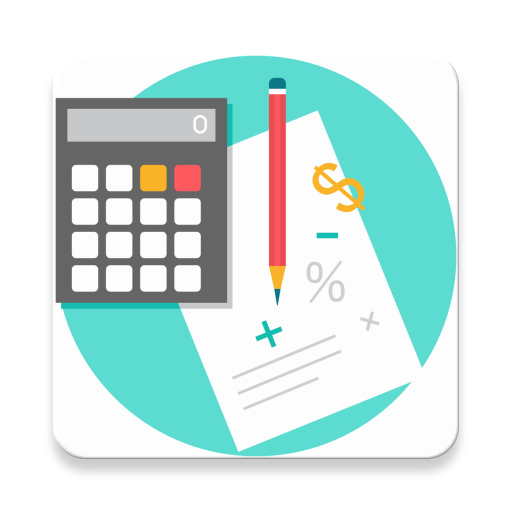#### Current Version : V1.1 Downloads : 809 Size : 2.4 MB

Body Calculator
- BMI (Body Mass Index) - Body Mass Index (BMI) I a measure of body fat based on height and weight that applies to adult men and women. BMI can be used to indicate if you are overweight, obese, underweight or normal. A score below 20 indicates that you are underweight; while a score above 25 means you may be overweight. Values are shown here fall into following categories:
- Underweight
- Normal Weight
- Overweight
- Obese (Class 1) - Moderately obese
- Obese (Class 2) - Severely obese
- Obese (Class 3) - Very Severely obese

- ABF (Army Body Fat) - Army Body Fat Calculator is based on the U.S Army Regulations of Standard Medical Fitness. This calculator will determine your body fat percentage.

- Calorie and Carbohydrate Intake - The Carbohydrate Calculator estimates the percentage of carbohydrates you will need each day. The Calorie Calculator estimates the number of calories a person need to consume each day.

- Body Fat - The Body Fat Calculator can be used to estimate your total body fat based on your size.

Math Calculator
- Fraction Calculator - Enter numbers of your choice and press “Calculate” button to get a result.
- Fraction Subtraction
- Fraction Multiplication
- Fraction Division
- Simplify Fraction
- Fraction to Decimal
- Decimal to Fraction

- Percentage Calculator
- Calculate Percentage
- Percentage Difference
- Percentage Increase
- Percentage Decrease

- Error Find Calculator
- Absolute Error
- Relative Error
- Percent Error

- Exponent Calculator -

- Root Calculator

- Volume Calculator - Volume Calculator for various shapes available in app are:
- Calculate Ball Volume
- Calculate Cone Volume
- Calculate Cube Volume
- Calculate Rectangular Tank Volume
- Calculate Capsule Volume
- Calculate Cap Volume

- Ratio Calculator
- Area Calculator - Area Calculator for various shapes to estimate area available in app are:
- Area of Rectangle
- Area of Square
- Area of Triangle
- Area of Circle
- Area of Trapezoid
- Area of Ellipse

- GCD Calculator

- LCM Calculator

ASWDC is established by Department of Computer Engineering where students work on live projects under guidance of staff and industry experts. Students are getting extensive knowledge and industrial experience of cutting edge technologies. ASWDC fills gap between academic curriculum and industry expectation.